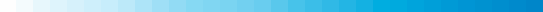Georgia Aeromagnetic Data ProcessingThe assembly of the 14 individual aeromagnetic surveys and grids to create the Georgia State-wide compilation was done in several steps.

GENERAL DATA PROCESSING STEPS (not all steps were applied to this State)

1. Grids were constructed from the original aeromagnetic survey data with a cell size of between 1/3 and 1/5 of the flightline spacing of the survey, using a minimum curvature gridding algorithm. For digitized contour line data, the initial grid was constructed using a minimum curvature algorithm and a spacing appropriate for the scale of the digitized map.
2. The Definitive Geomagnetic Reference Field (DGRF) calculated for the date and elevation of the original survey was subtracted (in some cases this required the determination and removal of the original reference field applied).
3. Flightlines were adjusted and spurious anomalies (generated by equipment malfunction) were removed as necessary.
4. Strike filtering in the direction of the flightlines was applied as necessary.
5. The survey grids were regridded, as necessary, to the final grid cell size of 500 m using a minimum curvature algorithm.
6. The original survey grids were continued to 1000 ft above ground, if necessary, and converted from level to drape as necessary. Upward continuation of the NURE surveys was by standard 2D FFT filtering techniques.
7. The datum levels of the converted grids were adjusted (by addition or subtraction of a constant value) to minimize differences at the boundaries.
8. These adjusted grids were combined into a single merged grid that shows the magnetic field measured or calculated at 1000 ft above ground.  Weighted averaging of the data was applied in the overlap areas.

GRID PROJECTION SPECIFICATIONS

• Projection = Lambert conformal conic
• Central meridian = 81 degrees W
• Base latitude = 0 degrees N
• Standard parallels = 33 and 45 N
• Semi-major ellipsoid axis = 6378206.4 m
• Eccentricity squared = 0.0067686579973

U.S. Department of the Interior, U.S. Geological Survey